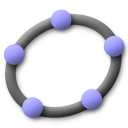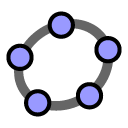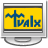GeoGebra

6.0.680

GeoGebra is a mathematical tool that can solve equations, graph functions, create constructions, analyze data, and create 3D plots. It has a simple interface which lets you construct and analyze polygons, conic sections, and other shapes using your mouse. Support for touch-screen interface makes it easier to work with graphs.

3.8
Rated by
293 users
Freeware
OS:
Windows 7/Vista/8/8.1/10
Date:
File size:
94.26 MB
Category rank:
3
All software rank:
241

Get GeoGebra v6.0.389; you will need 40.16 MB of free space on the hard drive; GeoGebra Geometry and GeoGebra Pre-Release are its known alternative names. This program is provided at no cost by its creator GeoGebra Inc. It is in the Education category, Science with .ggb formats support. Versions 6.0 and 5.0 are still the most popular ones and can be run on Windows 7/10. The most popular installers for the software are GeoGebra.exe and GeoGebra-JOGL2.exe. A number of antivirus services checked the file and found it safe to use.Download
GeoGebra 6.0.680

Thank you for rating the program!

Useful softwareSmart Math Calculator
Define variables and solve mathematical equations and functions.GeoGebra Classic
A collection of mathematical tools for algebra, calculus and geometry.Math Calculator
It enables you to calculate expressions and solve equations.Math Center Level 1
The program helps you study graphics, make calculations and solve equations.Abacus Math Writer
For publishing Mathematical and Scientific equations.